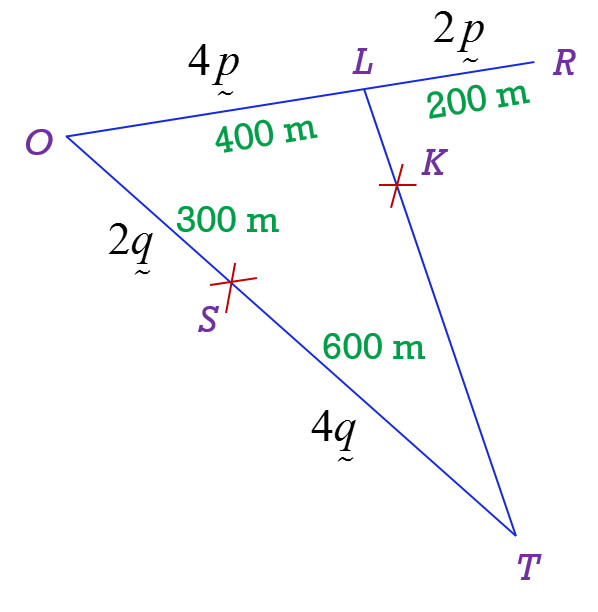\

# SPM Additional Mathematics 2019, Paper 2 (Question 6)

Question 6:
Solution by scale drawing is not accepted.
Diagram 3 shows the positions of jetty O and kelongs K, L, R, S and T in the sea.Diagram 3

Kelong
L is situated 400 m from jetty O and kelong R is situated 600 m from jetty O in the direction of OL. Kelong S is situated 300 m from jetty O and kelong T is situated 600 m from kelong 5 in the directions of OS. Kelongs L, K and T are situated on a straight line such that the distance of kelong K from kelong T is 5 times its distance from kelong L.

(a) By using to represent 100 m in the direction of OR and  to represent 150 m in the direction of OT, express in terms of
[3 marks]

(b) If Joe uses a binocular to observe kelong
R from kelong S, determine whether kelong R can be seen without being blocked by kelong K or otherwise.
Prove you answer mathematically.     [5 marks]

Solution:
(a)(a)(i)

(a)(ii)

(b)

S
, K and R are not collinear.
Hence, Kelong R can be seen without being blocked by Kelong K.